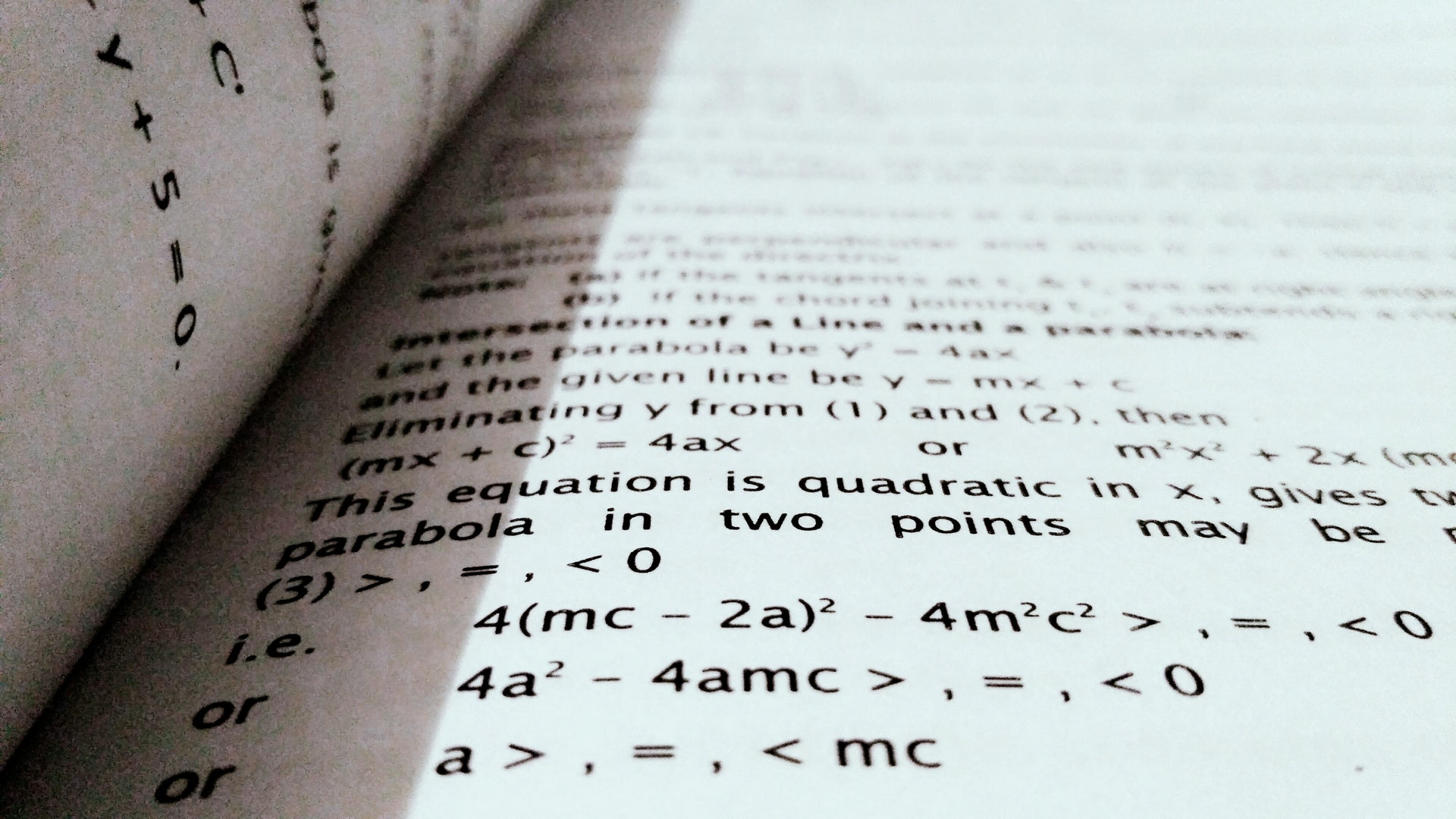# Using Sympy for Analytical Maths### This is an example of how i would use sympy to evaluate a set of analytical questions, for example:

Find $$\forall a \in [0,12,24,30,99]$$ and $$b=100$$:

Where $$\alpha = 2.341$$, $$\beta = e^x$$

First we need to import the library (and start the printing for nice easy to read stuff):

import sympy as sp
sp.init_printing()


Now create the eqn above so we can evaluate it

a, b, alpha, beta, x = sp.symbols('a b \\alpha \\beta x')

expr = (alpha*x**3 - sp.sin(2*x)) * (1/sp.sqrt(beta*sp.E**x))
expr


This like the integrand we hav above. Now theres a few things we can do, if we know $$\alpha$$ and $$\beta$$, which we do, we can sub them in. then we will have a more simple expression:

expr2 = expr.subs({alpha: 2.341, beta:sp.E**x})
expr2


If we wanted we could now evaluate this using the .subs() command again (this time with a value for x such as .subs(x,3)), but we’re asked to evalue the integral:

integral = sp.integrate(expr2, (x,a,b))
integral


Now we need to calculate all the values of this expression for every combination of $$a \in [0,12,24,30,99]$$ and $$b=100$$. We can do this by starting with lambdifying the expression into a callable function:

my_callable = sp.lambdify((a, b), integral)
my_callable(1,2) # this will sub in a=1 and b=2 to the above.


Now we just loop over the varable s for a and we can print out the values:

for a in [0,12,24,30,99]:
print("a =",a)
print(my_callable(a,100))
print()

a = 0
13.645999999999999

a = 12
0.03219056961943131

a = 24
1.3876938438535285e-06

a = 30
6.546926902186657e-09

a = 99
1.470461229758446e-37


This decreasing sequence is expected as were taking essentially taking area slices of the below plot, moving further left each time as a starting point.

%matplotlib inline
import matplotlib.pyplot as plt
X = [x for x in range(0,101)]
expr2_callable = sp.lambdify(x, expr2)
plt.plot(X,[expr2_callable(x) for in_x in X])

[<matplotlib.lines.Line2D at 0x24a166e8668>]